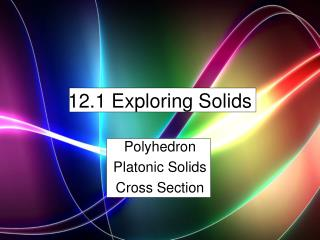DownloadDownload Presentation12.1 Exploring Solids

# 12.1 Exploring Solids

Download Presentation## 12.1 Exploring Solids

- - - - - - - - - - - - - - - - - - - - - - - - - - - E N D - - - - - - - - - - - - - - - - - - - - - - - - - - -
##### Presentation Transcript

1. 12.1 Exploring Solids Polyhedron Platonic Solids Cross Section

2. Definition of a Polyhedron A polyhedron is a solid formed by many plane faces.

3. Convex Polyhedron Convex Polyhedron are polyhedrons where any two points can be connected by a line segment

4. Faces, Edges and Vertices A Cube has 6 Faces, 12 Edges and 8 Vertices.

5. Cross section The cutting of a polyhedron or cone by a plane giving different shapes.

6. Regular Polyhedron A regular polyhedron has regular polygons for faces

7. Platonic Solids are regular polyhedrons

8. Can you think of any use of a Icosahedrons?

9. Euler’s Theorem The number of faces + number of vertices equals the number of edges plus 2. Icosahedrons has 20 faces, 12 vertices. How many Edges?

10. Euler’s Theorem The number of faces + number of vertices equals the number of edges plus 2. Icosahedrons has 20 faces, 12 vertices. How many Edges?

11. How many Edges on this shape? Edge = ½(Shape edges times Number of Shapes + Shape edges times Number of Shapes…..)

12. How many Edges on this shape? Edge = ½ (8 sides* 6 + 4 sides* 10 + 6 sides * 8)

13. How many Edges on this shape? Edge = 68 ½ (8 sides* 6 + 4 sides* 10 + 6 sides * 8)

14. How many Vertices on this shape? Edge = 68, Faces = (6 +10 + 8) = 24

15. How many Vertices on this shape? Edge = 68, Faces = (6 +10 + 8) = 24 24 + V = 68 + 2 24 + V = 70 V = 46

16. Homework Page 723 – 726 # 10 – 30 even, 32 – 35 , 42- 52, 54, 55